# Clocks

What distance will describe the tip of minute hand 6 cm long for 20 minutes when we know the starting position with finally enclose hands each other 120°?

x =  12.57 cm

### Step-by-step explanation: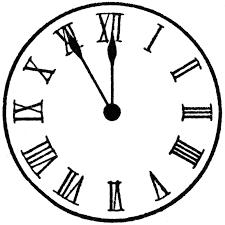Did you find an error or inaccuracy? Feel free to write us. Thank you!Tips to related online calculators
Do you want to convert length units?
Do you want to convert time units like minutes to seconds?

#### You need to know the following knowledge to solve this word math problem:

We encourage you to watch this tutorial video on this math problem:

## Related math problems and questions:

• Minute handWhat is the distance the minute hand of the clock travels in 12 minutes, if the diameter of the clock is 30 cm and the hand extends to a distance of 2 cm from the edge of the clock?
• Circular arcCalculate the length of the circular arc if the diameter d = 20cm and the angle alpha = 142 °
• Minute-hand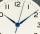How long distance will travel a large (minute) hand on the clock for 48 minutes, if its length is 56 cm?
• ClocksHow will long path walk the end of the clock's minute-hand long 7 cm for 330 minutes? How long will walk in the same time hour-hand?
• HandsThe clock shows 12 hours. After how many minutes will angle between the hour and minute hand 90°? Consider the continuous movement of both hands hours.
• ArcCircle arc corresponding to angle is 32° is 28 dm long. What is the length of the entire circle?
• Special watchFero bought a special watch on the market. They have only one (minute) hand and a display that shows which angle between the hour and minute hand. How many hours was his watch shown - the minute hand points to number 2; the display shows 125°?
• The length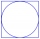The length of the circle is 24 cm. What is the length of the circular arc of the corresponding angle 30°?
• Internal anglesThe ABCD is an isosceles trapezoid, which holds: |AB| = 2 |BC| = 2 |CD| = 2 |DA|: On its side BC is a K point such that |BK| = 2 |KC|, on its side CD is the point L such that |CL| = 2 |LD|, and on its side DA the point M is such that | DM | = 2 |MA|. Dete
• WalkersWalker, which makes 120 steps per minute, make distance from point A to point B for 55 minutes. The length of his step is 75cm. For how long does this distance go boy who will do 110 steps 60 cm long in a minute?
• ClocksThe length of the minute hand is 12 cm. What track in meters will its end point describe in a week?
• The big clock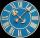The big clock hands stopped at a random moment. What is the probability that: a) a small hand showed the time between 1:00 and 3:00? b) the big hand was in the same area as a small hand in the role of a)? c) did the hours just show the time between 21:00
• Length of the arcWhat is the arc length of a circle k (S, r=68mm), which belongs to a central angle of 78°?
• Chord MNChord MN of circle has distance from the center circle S 120 cm. Angle MSN is 64°. Determine the radius of the circle.
• Clock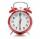What distance will pass end of 8 cm long hour hand for 15 minutes?
• ClocksHow long track travel the second hand of a clock in 46 hours, which is 4 cm long.
• Pendulum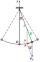Calculate the pendulum's length that is 2 cm lower in the lowest position than in the highest position. The length of the circular arc to be described when moving is 20cm.